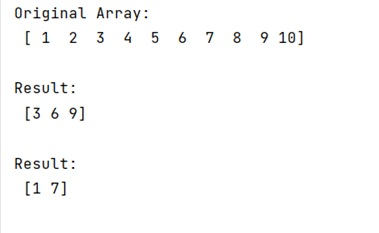# What is double colon (::) in NumPy like in arr[0::3]?

Learn about the use of double colon (::) in NumPy like in arr[0::3] in Python.
Submitted by Pranit Sharma, on February 28, 2023

NumPy is an abbreviated form of Numerical Python. It is used for different types of scientific operations in python. Numpy is a vast library in python which is used for almost every kind of scientific or mathematical operation. It is itself an array which is a collection of various methods and functions for processing the arrays.

## Use of double colon (::) in NumPy

Slicing in Python is a feature that enables accessing parts of sequences like strings, tuples, and lists. We can also use them to modify or delete the items of mutable sequences such as lists.

For example, if we have an array [1,2,3,4,5,6,7,8,9,10], then we need to understand what would be the output if we use array[0::3].

This type of slicing technique is used to print every kth element from the list/array.

The additional syntax of a[x : : k] means to get every kth element starting at position x.

Let us understand with the help of an example,

## Python code to demonstrate the use of double colon (::) in NumPy like in arr[0::3]

```# Import numpy
import numpy as np

# Creating a numpy array
arr = np.array([1,2,3,4,5,6,7,8,9,10])

# Display original image
print("Original Array:\n",arr,"\n")

# Using double colon
res = arr[2::3]

# Display result
print("Result:\n",res,"\n")

# Using double colon
res = arr[0::6]

# Display result
print("Result:\n",res,"\n")
```

Output:Languages: » C » C++ » C++ STL » Java » Data Structure » C#.Net » Android » Kotlin » SQL
Web Technologies: » PHP » Python » JavaScript » CSS » Ajax » Node.js » Web programming/HTML
Solved programs: » C » C++ » DS » Java » C#
Aptitude que. & ans.: » C » C++ » Java » DBMS
Interview que. & ans.: » C » Embedded C » Java » SEO » HR
CS Subjects: » CS Basics » O.S. » Networks » DBMS » Embedded Systems » Cloud Computing
» Machine learning » CS Organizations » Linux » DOS
More: » Articles » Puzzles » News/Updates